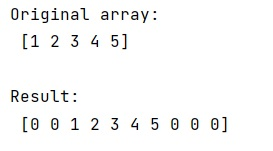Given a NumPy array, we have to pad it with zeros.
Submitted by Pranit Sharma, on February 10, 2023

NumPy is an abbreviated form of Numerical Python. It is used for different types of scientific operations in python. Numpy is a vast library in python which is used for almost every kind of scientific or mathematical operation. It is itself an array which is a collection of various methods and functions for processing the arrays.

## Padding NumPy Array with Zeros

Suppose that we are given with a numpy array and we need to pad this numpy array with zeroes. By padding, we mean that we need to insert zeroes in this numpy array up to some specific position.

Numpy provides a method called numpy.pad() which is used to pad an array. It takes an argument called pad_width.

These are the number of values padded to the edges of each axis.

• ((before_1, after_1), ... (before_N, after_N)) unique pad widths for each axis.
• (before, after) or ((before, after),) yields same before and after pad for each axis.
• (pad,) or int is a shortcut for before = after = pad width for all axes.

Let us understand with the help of an example,

## Python code to pad a NumPy array with zeros

```# Import numpy
import numpy as np

# Creating a numpy array
arr =  np.array([1, 2, 3, 4, 5])

# Display original array
print("Original array:\n",arr,"\n")

# Padding the array with zeroes
res = np.pad(arr, (2, 3), 'constant', constant_values=(0))

# Display Result
print("Result:\n",res,"\n")
```

Output: